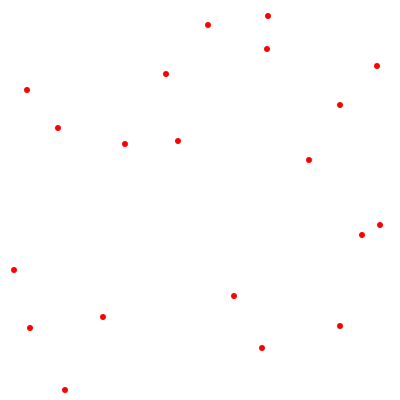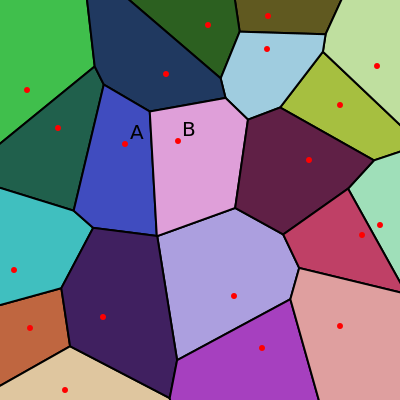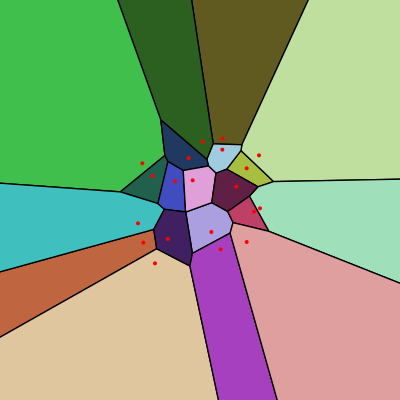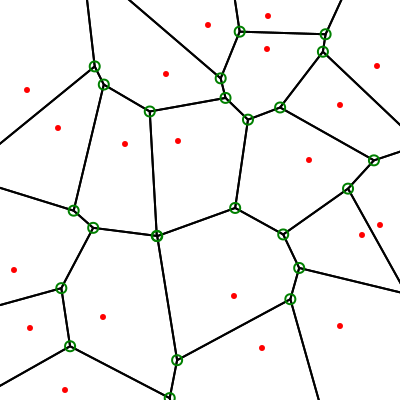# Voronoi diagrams with SciPy

Martin McBride
2021-06-25

In this post we will see how to create Voronoi diagrams in Python, using scipy and generativepy.

## What are Voronoi diagrams?

This image shows a set of seed points. We have placed the seed points randomly, but you could place the points according to some pattern if you prefer.A Voronoi diagram divides the plane into separate regions. Each region is associated with a particular seed point, and that region indicates the area that is closer to that seed point than any other seed point.

This diagram shows the regions, each filled with a different colour:So, for example, the seed point marked A in the diagram is surrounded by a blue region. This region contains all the points that are closer to the point A than any other seed point. Similarly, the seed point marked B in the diagram is surrounded by a light magenta region that contains all the points that are closer to point B than any other seed point.

The lines on the diagram indicate points that are an equal distance between two seed points. The places where two or more lines join are points that are an equal distance from 3 (or more) seed points.

One important point to note is that the regions around the edge of the cluster of points extend out to infinity. Here is a "zoomed out" version of the previous image:This makes sense, of course, because every point on the plane has to be closest to one (or more) of the seed points.

## Uses of Voronoi diagrams

Voronoi diagrams have lots of uses, particularly in predicting or simulating interactions between neighbouring structures. For example, modelling biological cell structures, modelling growth patterns in forests, estimating mineral reserves for mining, mapping the closest airfield for planes that need to land in an emergency, and many others.

In generative art, we might look at a couple of uses in future articles:

• Creating mandalas and other patterns by creating a Voronoi diagram based on a complex pattern of points.
• Creating cell-like art by eroding the regions in a Voronoi diagram.

## Creating Voronoi diagrams with SciPy and generativepy

Scipy uses the Voronoi class to create Voronoi diagrams.

First, we create a Voronoi object:

voronoi = Voronoi(points)


Here points is a list of points, where each point is a list of [x, y] values. For example:

points = [[234, 296], [268, 16], [125, 144], [340, 326] ...


The next step is to find the vertices of the diagram. These points represent the position of every vertex in the diagram. A vertex is any point where lines in the diagram meet. They are indicated by green circles in this image (remember, the red dots represent the seed points):We can get a list of the Voronoi vertices using this code:

voronoi_vertices = voronoi.vertices


The list might look something like this:

[[   70.07931175   346.25361434]
[  169.70719593   398.11470473]
[   61.32426304   288.15192744]
[  325.59158212    34.26035097]
...


This is actually a NumPy array, but you can index it just like a list.

Finally, we can get a list of the Voronoi regions, using this code:

regions = voronoi.regions


The result will be a list of lists, and it might look something like this:

[[17, 8, 9, 15], [16, 13, 6, 9, 15], [4, 0, -1, 1, 2, 3] ...


Here each sub-list represents a single region. The elements in the list are indices into the vertices array. So for example, the first region is given by:

[17, 8, 9, 15]


This list has length 4, which means the region has 4 vertices (so it is a quadrilateral). The first element is 17, so we can find the position of the first vertex by looking up voronoi_vertices. The second vertex is at voronoi_vertices, and so one. When we have looked up all 4 vertices, we can draw the quadrilateral. That will be one part of the diagram.

The second region is given by [16, 13, 6, 9, 15]. We can look up the position of these 5 vertices, and draw the shape (an irregular pentagon, in this case). If we repeat this to draw every region, we will have a complete diagram.

There is one extra thing we need to do. You will notice that the third region has -1 as one of its indices. What does that mean? Well, it indicates that it is one of the regions at the edge of the diagram, that extends out to infinity. The polygon goes includes vertices 4, 0, 1, 2, 3, but vertices 0 and 1 are not connected, the polygon extends forever.

Unfortunately, SciPy doesn't give us enough information to draw these lines. It is certainly possible to calculate them, but it requires extra code. But there is a useful hack to avoid this.

## Drawing the edge regions

The trick to drawing the edge regions is to add some extra distant points to the diagram. These points outside the visible area of the image.

The extra points create new edge regions. This means that the regions that were previously at the edges now become ordinary, closed regions that we can draw in the usual way. And the new edge regions are so far off the image that we can just ignore them, and not draw them at all.

It is sufficient to draw 4 distant points. Typically, we can place these on the 4 diagonals of the image, at a distance of several times the size of the image.

After adding these extra points, we can simply ignore any regions containing -1, because they are beyond the boundaries of the image.

## The code

Here is the basic code to create the diagram we saw at the start of this article:

from generativepy.drawing import make_image, setup
from generativepy.geometry import Circle, Polygon
from generativepy.color import Color
from scipy.spatial import Voronoi
import random

SIZE = 400
POINTS = 20

# Create a list of random points
random.seed(40)
points = [[random.randrange(SIZE), random.randrange(SIZE)]
for i in range(POINTS)]
points.append((-SIZE*3, -SIZE*3))
points.append((-SIZE*3, SIZE*4))
points.append((SIZE*4, -SIZE*3))
points.append((SIZE*4, SIZE*4))

def draw(ctx, pixel_width, pixel_height, frame_no, frame_count):
setup(ctx, pixel_width, pixel_height, background=Color(1))
voronoi = Voronoi(points)
voronoi_vertices = voronoi.vertices

for region in voronoi.regions:
if -1 not in region:
polygon = [voronoi_vertices[p] for p in region]
Polygon(ctx).of_points(polygon).stroke(line_width=2)

make_image("voronoi-lines.png", draw, SIZE, SIZE)


We will go through this, step by step.

After importing some generativepy and SciPy modules, define some constants. SIZE is the output image size, we will make the image 400 by 400 pixels. POINTS is the number of points in the diagram.

Next we create some random points:

# Create a list of random points
random.seed(40)
points = [[random.randrange(SIZE), random.randrange(SIZE)]
for i in range(POINTS)]
points.append((-SIZE*3, -SIZE*3))
points.append((-SIZE*3, SIZE*4))
points.append((SIZE*4, -SIZE*3))
points.append((SIZE*4, SIZE*4))


We first set the random seed to 40. This ensures that we will always get the same set of points each time we run the code, which is useful if you want to try different things out by running the code several times with the same points. If you want to create a different set of points, change the 40 to any other number (or comment that line out so you will get a different set of points every time the code runs).

We then use a list comprehension to create a list of 2 points with x and y coordinates in the range 0 to SIZE-1.

Finally, we add four distant points that are well outside the image in the direction of each diagonal.

We are using generativepy to do our drawing. This requires us to define a draw function that takes several fixed parameters - a drawing context ctx, the width and height of the image in pixels, and a couple of others we will ignore (they are only used for animations, not still images).

The draw function is responsible for drawing the image. The first thing we do is call the setup function, which sets the background colour to white.

def draw(ctx, pixel_width, pixel_height, frame_no, frame_count):
setup(ctx, pixel_width, pixel_height, background=Color(1))
voronoi = Voronoi(points)
voronoi_vertices = voronoi.vertices

for region in voronoi.regions:
if -1 not in region:
polygon = [voronoi_vertices[p] for p in region]
Polygon(ctx).of_points(polygon).stroke(line_width=2)


Inside the draw function, we use a Voronoi object to find the vertices as described previously.

Then we loop over the regions. If the region contains a value of -1, we ignore that region

For valid regions, we create a list polygon by looking up the vertex coordinates for each index in the region. Then we draw the polygon using the generativepy Polygon class.

Finally, we call the generativepy make_image function, which calls the draw function to draw the image, and writes the result to a PNG file.

make_image("voronoi-lines.png", draw, SIZE, SIZE)